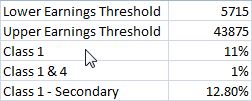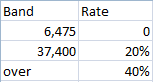## Calculating National InsuranceCreate the cells above and create names for each of the values from the names in the left column.

Two functions are available, one for employee national insurance, one for employer.

The calls are

=NationalInsurance(50000.0,Lower_Earnings_Threshold,Upper_Earnings_Threshold,Class_1,Class_1___4)

=EmployerNationalInsurance(50000.0,Lower_Earnings_Threshold,Class_1___Secondary)

The code for these functions is this

`Function NationalInsurance( _   income As Double, _   lowerearnings As Double, _   upperearnings As Double, _   classonerate As Double, _   secondaryrate As Double _   ) As Double   NationalInsurance = 0#   If income <= lowerearnings Then               Exit Function       End If       If income <= upperearnings Then               NationalInsurance = (income - lowerearnings) * classonerate       Else               NationalInsurance = (upperearnings - lowerearnings) * classonerate + (income - upperearnings) * secondaryrate       End If     End Function Function EmployerNationalInsurance( _   income As Double, _   lowerearnings As Double, _   secondaryrate As Double _   ) As Double   EmployerNationalInsurance = 0#   If income <= lowerearnings Then       Exit Function   End If   EmployerNationalInsurance = (income - lowerearnings) * secondaryrateEnd Function`

## Calculating UK TaxWith this as a table, the following function call can be made from Excel

=IncomeTax(50000.0,TaxRates)

To calculate the tax paid. TaxRates is the range above.

The code for this is as follows.

`Function IncomeTax(income As Double, rates As Range) As Double    Dim i As Integer    Dim tax As Double    tax = 0    For i = 1 To rates.Rows.Count - 1        tax = tax + Application.Min(income, rates.Cells(i, 1)) * rates.Cells(i, 2)        income = Application.Max(0, income - rates.Cells(i, 1))    Next i    IncomeTax = tax + income * rates.Cells(rates.Rows.Count, 2)End Function`Subscribe to Posts [Atom]